# R S Aggarwal Solutions for Class 10 Maths Chapter 4 Quadratic Equations

R S Aggarwal Solutions for Class 10 Chapter 4 Quadratic Equations is a set of important questions which helps students to master quadratic equations. These solutions help students in preparing for the exams in a better way. Download RS Aggarwal Class 10 Maths Solutions for Chapter 4. Basically, this study material is aimed at mastering the concepts and acquiring comprehensive knowledge about the varied types of questions asked in CBSE Class 10 Maths exams.

### Access Answers to Maths R S Aggarwal Chapter 4 Quadratic Equations

Get detailed solutions for all the questions listed under the below exercises:

Exercise 4A Solutions: 30 Questions (Short Answers)

Exercise 4B Solutions: 16 Questions (Short Answers)

Exercise 4C Solutions: 15 Questions (Short Answers)

Exercise 4D Solutions: 20 Questions (Long Answers)

## Exercise 4A Page No: 175

Question 1:

Solution:

A quadratic equation is an equation of the second degree.

(i) x2 – x + 3 = 0

Highest Degree: 2

(ii) 2x2 + 5/2x – √3 = 0

Highest Degree: 2

(iii) √2x2+7x+5√2=0

Highest Degree: 2

(iv) 1/3x2 + 1/5x – 2 = 0

Above equation can be simplify as: 5x2 + 3x – 2 = 0

Highest Degree: 2

(v) x2 – 3x – √x + 4 = 0

Equation has a fractional power.

(vi) x – 6/x = 3

Simply as x2 – 3x – 6 = 0

Degree : 2

(vii) x + 2/x = x2

Simplify above equation:

x3 – x2 – 2 = 0

Degree: 3

(viii) x^2 – 1/x^2 = 5

Simplify above equation

x4   – 1 = 5 x2

or x4-5x2-1 =0

Degree: 4

(ix) (x + 2)^3 = x^3 – 8

x^3 + 8 + 6x^2 + 12x = x^3 – 8

-6x^2 + 12x + 16 = 0

Degree = 2

(x) (2x + 3)(3x + 2) = 6(x – 1)(x – 2)

Simplify above equation:

6x^2 + 4x + 9x + 6 = 6x^2 – 12x – 6x + 12

31x – 6 = 0

Degree : 1

(xi) (x + 1/x)^2 = 2)x + 1/x) + 3

Simplify above equation:

(x^4 + 2x^2 + 1) / x^2 = (2x^2 + 2) / x + 3

(x^4 + 2x^2 + 1)x = x^2(2x^2 + 2) + 3

Answer: (i), (ii), (iii), (iv), (vi) and (ix) are only quadratic equations.

Question 2:

Solution:

Simplify given equation:

3x² + 2x – 1 = 3x² + 3x – x – 1

= 3x (x + 1) – 1 (x + 1)

= (x + 1) (3x – 1)

To find roots, put 3x² + 2x – 1 = 0

Either, x + 1 = 0 or 3x – 1 =0

x = -1 or x = 1/3

Therefore, (-1) and 1/3 are the required roots.

Question 3:

Solution:

(i) x = 1 is a solution of x^2+kx+3=0, which means it must satisfy the equation.

(1)^2 + k(1) + 3 = 0

k = -4

Hence the required value of k = -4

Find other root:

We have equation, x^2 – 4x + 3 = 0

x^2 – x – 3x + 3 = 0

x(x – 1) – 3(x – 1) = 0

(x – 1)(x – 3) = 0

either x – 1 = 0 or x – 3 = 3

x = 1 or x = 3

Other root is 3.

(ii) given equation is ax² + bx – 6 = 0

As ¾ is its root, then must satisfy the equation

a(3/4)² + b(3/4) – 6 = 0

9a +12b – 96 = 0 ….(1)

Again, x = -2 is its root

a(-2)² + b(-2) – 6 = 0

4a – 2b – 6 = 0 …(2)

Solving (1) and (2), we get

a = 4 and b = 5

Question 4: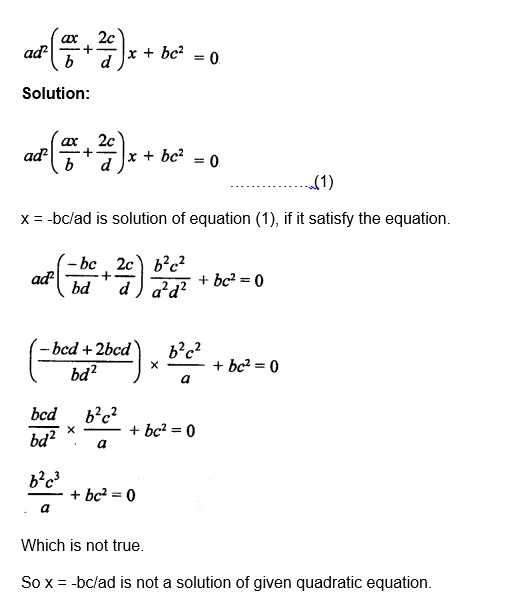Question 5:

Solution:

(2x – 3)(3x + 1) = 0

Either 2x – 3 = 0 or 3x + 1 = 0

x = 3/2 or x = -1/3

Question 6:

Solution:

4x2 + 5x = 0

Or x (4x + 5) = 0

Either x = 0 or 4x + 5 = 0, then

x = -5/4 or 0

Question 7:

Solution:

3x² – 243 = 0

or x² – 81 = 0

(x)² – (9)² = 0

(x + 9) (x – 9) = 0

Either, x + 9 = 0 or x – 9 = 0

x = -9 or 9

Question 8:

Solution:

2x2 + x – 6 = 0

2x2 + 4x – 3x – 6 = 0

2x (x + 2) – 3(x + 2) = 0

(x + 2)(2x – 3)= 0

Either x + 2 = 0 or 2x – 3 = 0

x = -2 or 3/2

Question 9:

Solution:

x^2 + 6x + 5 = 0

x^2 + x + 5x + 5 = 0

x(x + 1) + 5(x + 1) = 0

(x + 5)(x + 1) = 0

either x +5 = 0 or x + 1 = 0

x = -5 or -1

Question 10:

Solution:

9x^2 – 3x – 2 = 0

9x^2 – 6x + 3x – 2 = 0

3x(3x -2) + (3x – 2) = 0

(3x +1)(3x – 2) = 0

either (3x +1) = 0 or (3x – 2) = 0

x = -1/3 or 2/3

Question 11:

Solution:

x² + 12x + 35 = 0

x² + 7x + 5x + 35 = 0

x(x + 7) + 5((x + 7) = 0

(x + 5)(x + 7) = 0

either (x + 5) = 0 or (x + 7) = 0

x = -5 or -7

Question 12:

Solution:

x^2 – 18x + 77 = 0

x^2 – 7x – 11x + 77 = 0

x (x – 7) – 11(x – 7) = 0

(x – 11)(x – 7) = 0

either (x – 11) = 0 or (x – 7) = 0

x = 11 or 7

Question 13:

Solution:

6x^2 + 11x + 3 = 0

6x^2 + 2x + 9x + 3 = 0

2x(3x + 1) + 3(3x + 1) = 0

(2x + 3)(3x + 1) = 0

either (2x + 3) = o or (3x + 1) = 0

x = -1/3 or -3/2

Question 14:

Solution: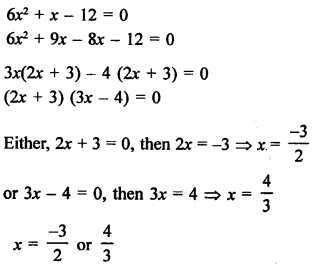Question 15:

Solution: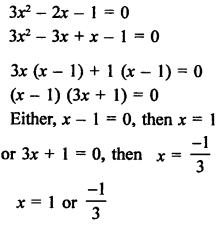Question 16:

Solution:

4x² – 9x = 100

4x² – 9x – 100 = 0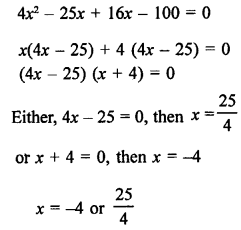Question 17:

Solution:

15x2  28  x

15x2  x  28  0

15x2  21x  20x 28  0

15x2  21x  20x  28  0

3x 5x  7  4 5x  7  0

3x  45x  7  0

3x  4  0 or 5x  7  0

x = -4/3 or 7/5

Question 18:

Solution:

4 11x  3x2

3x2 11x  4  0

3x2 12x x  4  0

3x x  4 1x  4  0

x  43x 1  0

Either x  4  0 or 3x 1  0

x = -4 or 1/3

Question 19:

Solution:

48x2 13x 1  0

48x2 16x  3x1  0

48x2 16x  3x 1  0

16x3x 113x 1  0

16x 13x 1  0

Either 16x 1  0 or 3x 1  0

x = -1/16 or 1/3

Question 20:

Solution: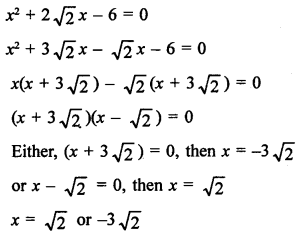Question 21;

Solution:

√3 x² + 10x + 7√3 = 0

√3 x² + 10x + 7√3 = 0

√3 x² + 3x + 7x + 7√3 = 0

√3 x(x + √3) + 7( x + √3) = 0

(x + √3)(√3 x + 7) = 0

either √3 x + 7 = 0 or x + √3 = 0

x = -√3 or -7/√3

Question 22:

Solution:

√3 x² + 11x + 6√3 = 0

√3 x² + 9x + 2x + 6√3 = 0

√3 x (x + 3√3) + 2(x + 3√3) = 0

(√3x + 2)(x + 3√3) = 0

either (√3x + 2) = 0 or (x + 3√3) = 0

x = -3√3 or -2√3/3

Question 23:

Solution:

3√7 x² + 4x – √7 = 0

3√7 x² + 4x – √7 = 0

3√7 x² -3x + 7x – √7 = 0

3x(√7x – 1) + √7(√7x – 1) = 0

(3x + √7)(√7x – 1) = 0

either (3x + √7) = 0 or (√7x – 1) = 0

x = -√7/3 or 1/√7

Question 24:

Solution:

√7 x² – 6x – 13√7 = 0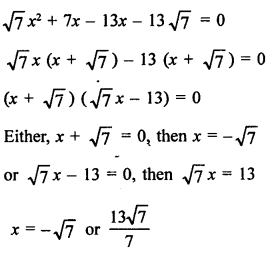Question 25:

Solution: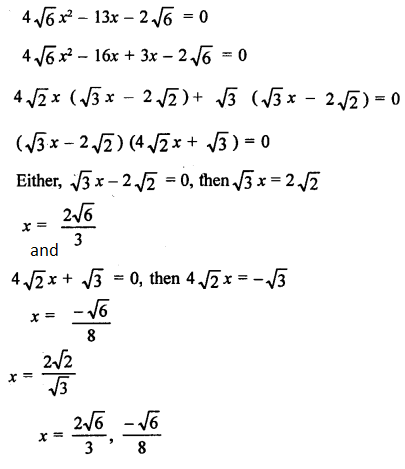Question 26:

Solution:

3x² – 2√6x + 2 = 0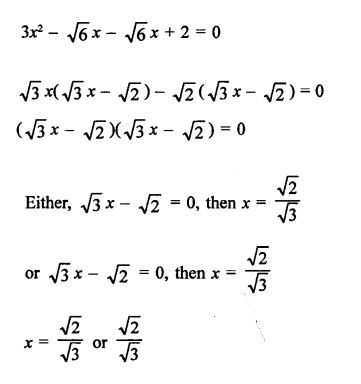Question 27:

Solution:

√3 x² – 2√2 x – 2√3 = 0

√3 x² – 3√2 x + √2 x– 2√3 = 0

√3x (x – √6) + √2(x – √6) = 0

(√3x+ √2)(x – √6) = 0

either (√3x+ √2) = 0 or (x – √6) = 0

x = √6 or -√2/√3

Question 28:

Solution: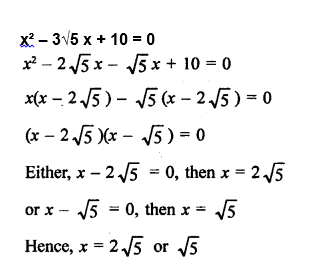Question 29:

Solution:

x² – (√3 + 1) x + √3 = 0

x² – (√3 + 1) x + √3 = 0

x² – √3x – x + √3 = 0

x(x – √3) – (x – √3) = 0

(x – 1)(x – √3) = 0

either (x – 1) = 0 or (x – √3) = 0

x = 1 or √3

Question 30:

Solution:

x² + 3√3 x – 30 = 0

x² + 5√3 x – 2 √3 – 30 = 0

x(x + 5√3) – 2√3 (x + 5√3) = 0

(x – 2√3)(x + 5√3) = 0

either (x – 2√3) = 0 or (x + 5√3) = 0

x = -5√3 or 2 √3

## Exercise 4B Page No: 185

Solve each of the following equations by using the method of completing the square:

Question 1:

Solution:

x² – 6x + 3 = 0

x^2 – 6x + 3 = 0

x^2 – 6x = – 3

x^2 – 2(x)3 + 3^2 = -3 + 3^2

(x – 3)^2 = – 3 + 9 = 6

Using algebraic identity: a^2 – 2ab + b^2 = (a – b)^2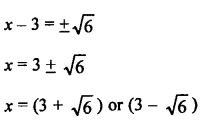Question 2:

Solution:

x^2 – 4x + 1 = 0

x^2 – 4x = – 1

x^2 – 2(x)(2) + 2^2 = – 1 + 2^2

(x – 2)^2 = 3

Using algebraic identity: a^2 – 2ab + b^2 = (a – b)^2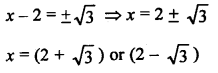Question 3:

Solution:

x^2 + 8x – 2 = 0

x^2 + 8x = 2

x^2 – 2.x.4 + 42 = 2 + 42

(x + 4)^2 = 18

Using algebraic identity: a^2 – 2ab + b^2 = (a – b)^2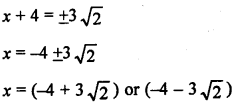Question 4:

Solution: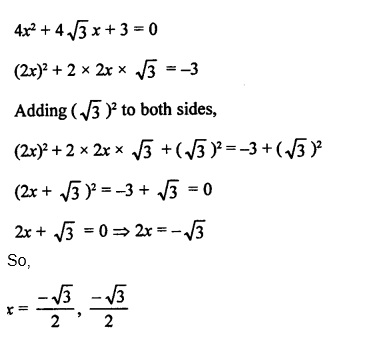Question 5:

Solution:

2x^2 + 5x – 3 = 0

4x^2 + 10x – 6 = 0

(multiplying both sides by 2)

4x^2 + 10x = 6

(2x + 5/2)^2 = 6 + 25/4 = 49/4

Using algebraic identity: a^2 – 2ab + b^2 = (a – b)^2

Taking square root,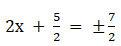2x + 5/2 = 7/2 or 2x + 5/2 = -7/2

x = 1/2 or -3

Question 6:

Solution:

3x^2 – x – 2 = 0

9x^2 – 3x – 6 = 0

(multiplying both sides by 3)

9x^2 – 3x = 6

Adding (1/2)^2 on both the sides.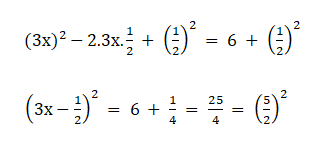3x – 1/2 = 5/2 or 3x – 1/2 = -5/2

x = 1 or x = -2/3

Question 7:

Solution:

8x^2 – 14x – 15 = 0

16x^2 – 28x – 30 = 0

(multiplying both sides by 2)

Adding (7/2)^2 on both the sides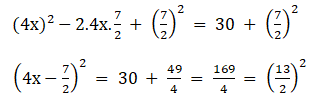4x – 7/2 = 13/2 or 4x – 7/2 = -13/2

x = 5/2 or x = -3/4

Question 8:

Solution:

7x^2 + 3x – 4 = 0

49x^2 + 21x – 28 = 0

(multiplying both sides by 7)

Adding (3/2)^2 on both the sides,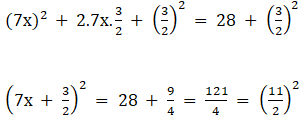7x + 3/2 = 11/2 or 7x + 3/2 = -11/2

x = -1 or x = 4/7

Question 9:

Solution:

3x^2 – 2x – 1 = 0

9x^2 – 6x = 3

(multiplying both sides by 3)

Adding (1)^2 on both the sides

(3x)^2 – 2.3x.1 + (1)^2 = 3 + (1)^2

(3x – 1)^2 = 2^2

3x – 1 = 2 or 3x – 1 = -2

x = -1 or x = -1/3

Question 10:

Solution:

5x^2 – 6x – 2 = 0

25x^2 – 30x – 10 = 0

(multiplying both sides by 5)

25x^2 – 30x = 10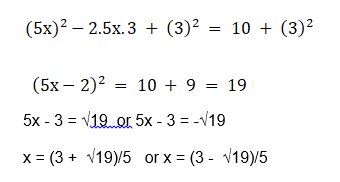Question 11:

Solution: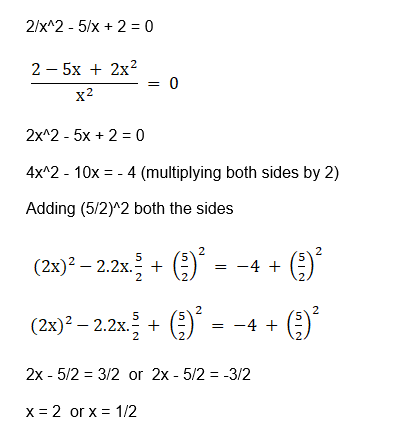Question 12:

Solution: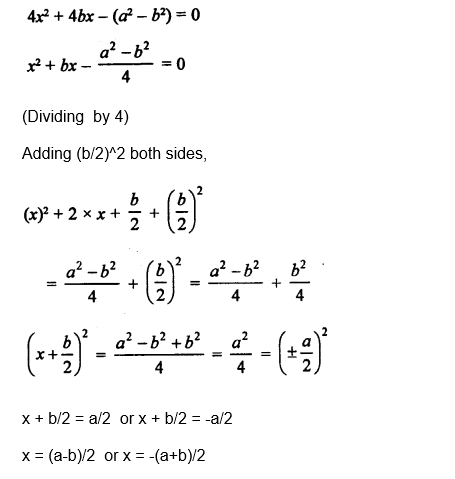Question 13:

Solution: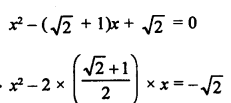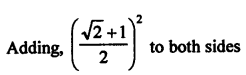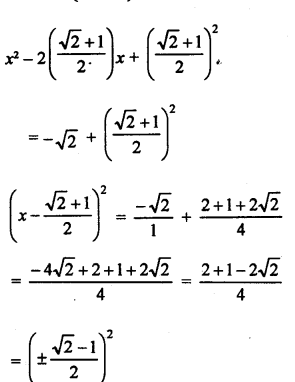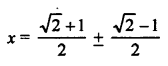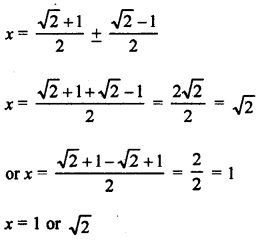Question 14:

Solution:

√2 x² – 3x – 2√2 = 0

Dividing each side by √2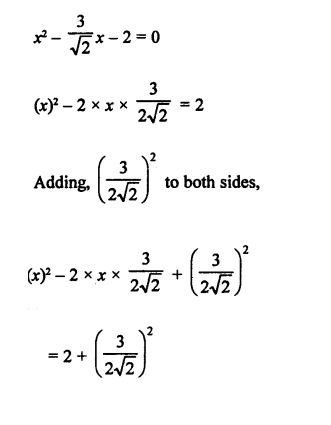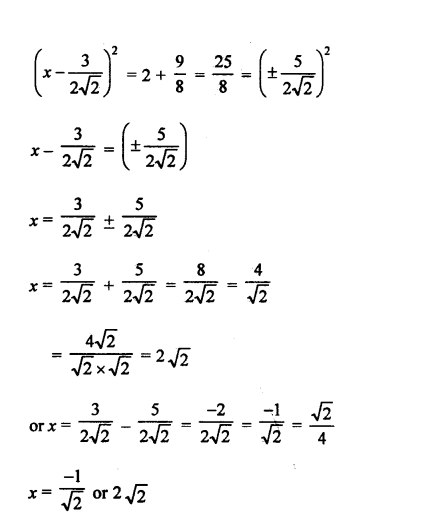Question 15:

Solution:

√3 x² + 10x – 7√3 = 0

Dividing each side by √3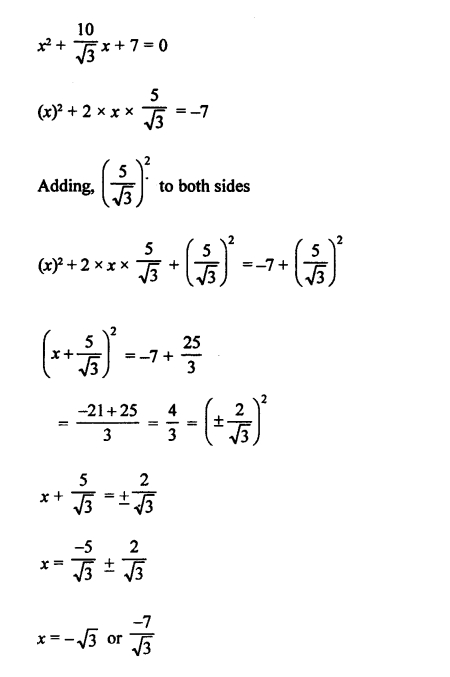Question 16:

Solution: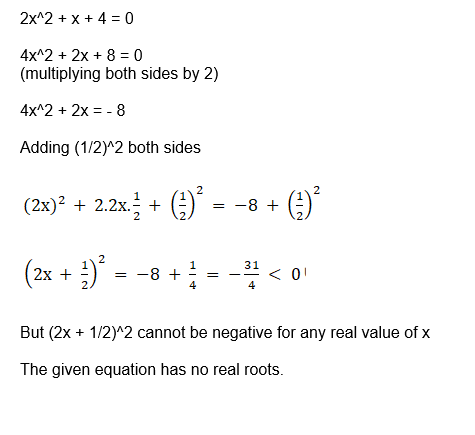## Exercise 4C Page No: 191

Find the discriminant of each of the following equations:

Question 1:

Solution:

(i) 2x² – 7x + 6 = 0

Compare given equation with the general form of quadratic equation, which is

ax^2 + bx + c = 0

Here, a = 2, b = -7 and c = 6

Discriminant formula: D = b^2 – 4ac

(-7)^2 – 4 x 2 x 6

= 1

(ii) 3x² – 2x + 8 = 0

Compare given equation with the general form of quadratic equation, which is ax^2 + bx + c = 0

Here, a = 3, b = – 2, c = 8

Discriminant formula: D = b^2 – 4ac

= (– 2)^2 – 4.3.8

= 4 – 96

= – 92

(iii) 2x² – 5√2x + 4 = 0

Compare given equation with the general form of quadratic equation, which is ax^2 + bx + c = 0

Here, a = 2, b = – 5√2, c = 4

Discriminant formula: D = b^2 – 4ac

= (– 5√2)^2 – 4.2.4

= 50 – 32

= 18

(iv) √3 x² + 2√2 x – 2√3 =0

Compare given equation with the general form of quadratic equation, which is ax^2 + bx + c = 0

Here, a = √3, b = 2√2, c = – 2√3

Discriminant formula: D = b^2 – 4ac

= (2√2)^2 – 4(√3)(– 2√3)

= 32

(v) (x – 1) (2x – 1) = 0

2x^2 – 3x + 1 = 0

Compare given equation with the general form of quadratic equation, which is ax^2 + bx + c = 0

Here, a = 2, b = -3, c = –1

Discriminant formula: D = b^2 – 4ac

= (-3)^2 – 4x2x1

= 1

(vi) 1 – x = 2x²

1 – x = 2x²

2x^2 + x – 1 = 0

Compare given equation with the general form of quadratic equation, which is ax^2 + bx + c = 0

Here, a = 2, b = 1, c = –1

Discriminant formula: D = b^2 – 4ac

= (1)^2 – 4x2x-1

= 9

Find the roots of each of the following equations, if they exist, by applying the quadratic formula:

Question 2:

Solution:

x² – 4x – 1 = 0

Compare given equation with the general form of quadratic equation, which is ax^2 + bx + c = 0

Here, a = 1, b = -4, c = –1

Find Discriminant:

D = b^2 – 4ac

= (-4)^2 – 4x1x-1

= 20 > 0

$\large x = \frac{-b\pm \sqrt{D}}{2a}$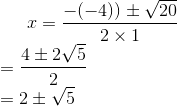Therefore, x = 2 + √5 and x = 2 – √5

Question 3:

Solution:

Compare given equation with the general form of quadratic equation, which is ax^2 + bx + c = 0

a = 1, b = -6, c = 4

Find Discriminant:

D = b^2 – 4ac

= (-6)^2 – 4.1.4

= 36 – 16

= 20 > 0

Roots of equation are real.

Find the Roots:

$\large x = \frac{-b\pm \sqrt{D}}{2a}$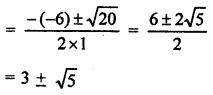x = 3 + √5 or x = 3 – √5

Question 4:

Solution:

Compare given equation with the general form of quadratic equation, which is ax^2 + bx + c = 0

a = 2, b = 1, c = -4

Find Discriminant:

D = b^2 – 4ac

= (1)^2 – 4.2.-4

= 1 + 32

= 33 > 0

Roots of equation are real.

$\large x = \frac{-b\pm \sqrt{D}}{2a}$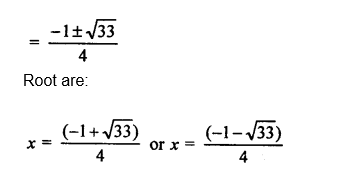Question 5:

Solution:

25x² + 30x + 7 = 0

Compare given equation with the general form of quadratic equation, which is ax^2 + bx + c = 0

a = 25, b = 30, c = 7

Find Discriminant:

D = b^2 – 4ac

= (30)^2 – 4.25.7

= 900 – 700

= 200 > 0

Roots of equation are real.

Find the Roots:

$\large x = \frac{-b\pm \sqrt{D}}{2a}$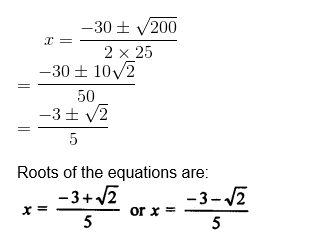Question 6:

Solution:

16x² – 24x – 1 =0

Compare given equation with the general form of quadratic equation, which is ax^2 + bx + c = 0

a = 16 , b = -24, c = -1

Find Discriminant:

D = b^2 – 4ac

= (-24)^2 – 4.16.-1

= 576 + 64

= 640 > 0

Roots of equation are real.

$\large x = \frac{-b\pm \sqrt{D}}{2a}$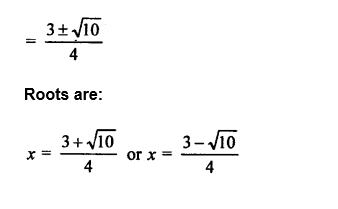Question 7:

Solution:

15x² -x – 28 = 0

Compare given equation with the general form of quadratic equation, which is ax^2 + bx + c = 0

a = 15, b =-1 , c = -28

Find Discriminant:

D = b^2 – 4ac

= (-1)^2 – 4.15.(-28)

= 1 + 1680

= 1681 > 0

Roots of equation are real.

$\large x = \frac{-b\pm \sqrt{D}}{2a}$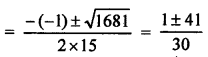X = 7/5 or x = -4/3

Question 8:

Solution:

2x² – 2√2 x + 1 = 0

Compare given equation with the general form of quadratic equation, which is ax^2 + bx + c = 0

a = 2, b = – 2√2, c = 1

Find Discriminant:

D = b^2 – 4ac

= (– 2√2)^2 – 4.2.1

= 8 – 8

= 0

Equation has equal root.

Find roots:

$\large x = \frac{-b\pm \sqrt{D}}{2a}$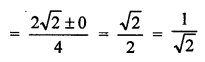x = 1/√2 or x = 1/√2

Roots of equation are real.

Question 9:

Solution:

√2 x² + 7x + 5√2 = 0

Compare given equation with the general form of quadratic equation, which is ax^2 + bx + c = 0

a = √2 , b = 7 , c = 5√2

Find Discriminant:

D = b^2 – 4ac

= (7)^2 – 4. √2 . 5√2

= 49 – 40

= 9> 0

Roots of equation are real.

Find roots:

$\large x = \frac{-b\pm \sqrt{D}}{2a}$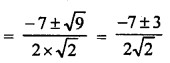Roots are:

x = – √2 or x = -5/√2

Question 10:

Solution:

√3 x² + 10x – 8√3 = 0

Compare given equation with the general form of quadratic equation, which is ax^2 + bx + c = 0

a = √3, b = 10 , c = – 8√3

Find Discriminant:

D = b^2 – 4ac

= (10)^2 – 4. √3 . – 8√3

= 100 + 96

= 196 > 0

Roots of equation are real.

Find roots:

$\large x = \frac{-b\pm \sqrt{D}}{2a}$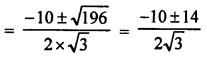Roots are:

x = 2√3/3 or x = -4√3

Question 11:

Solution:

√3 x² – 2√2 x – 2√3 = 0

Compare given equation with the general form of quadratic equation, which is ax^2 + bx + c = 0

a = √3 , b = – 2√2, c = – 2√3

Find Discriminant:

D = b^2 – 4ac

= (– 2√2)^2 – 4. √3 . – 2√3

= 8 + 24

= 32 > 0

Roots of equation are real.

Find roots:

$\large x = \frac{-b\pm \sqrt{D}}{2a}$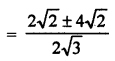x = √6 or x = -√2/√3

Question 12:

Solution:

2x² + 6√3 x – 60 = 0

Compare given equation with the general form of quadratic equation, which is ax^2 + bx + c = 0

a = 2, b = 6√3, c = – 60

Find Discriminant:

D = b^2 – 4ac

= (6√3)^2 – 4.2. – 60

= 108 + 480

= 588 > 0

Roots of equation are real.

Find roots:

$\large x = \frac{-b\pm \sqrt{D}}{2a}$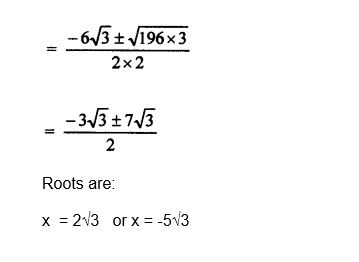Question 13:

Solution:

4√3 x² + 5x – 2√3 = 0

Compare given equation with the general form of quadratic equation, which is ax^2 + bx + c = 0

a = 4√3 , b = 5, c = – 2√3

Find Discriminant:

D = b^2 – 4ac

= (5)^2 – 4. 4√3 . – 2√3

= 25 + 96

= 121 > 0

Roots of equation are real.

Find roots:

$\large x = \frac{-b\pm \sqrt{D}}{2a}$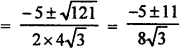Roots are:

x = √3/4 or x = -2/√3

Question 14:

Solution:

3x² – 2√6 x + 2 = 0

Compare given equation with the general form of quadratic equation, which is ax^2 + bx + c = 0

a = 3, b = – 2√6 , c = 2

Find Discriminant:

D = b^2 – 4ac

= (– 2√6)^2 – 4. 3 . 2

= 24 – 24

= 0

Roots of equation are equal.

Find roots:

$\large x = \frac{-b\pm \sqrt{D}}{2a}$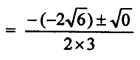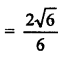Roots are:

x = √2/√3 and x = x = √2/√3

Question 15:

Solution:

2√3 x² – 5x + √3 = 0

Compare given equation with the general form of quadratic equation, which is ax^2 + bx + c = 0

a = 2√3, b = – 5 , c = √3

Find Discriminant:

D = b^2 – 4ac

= (– 5)^2 – 4. 2√3 . √3

= 25 – 24

= 1 > 0

Roots of equation are real.

Find roots:

$\large x = \frac{-b\pm \sqrt{D}}{2a}$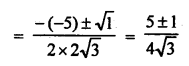Roots are:

x = √3/2 and x = 1/√3

## Exercise 4D Page No: 199

Question 1:

Solution:

(i)2x² – 8x + 5 = 0

Compare given equation with the general form of quadratic equation, which is ax^2 + bx + c = 0

a = 2, b = – 8, c = 5

Using Discriminant Formula:

D = b^2 – 4ac

= (– 8)^2 – 4.2.5

= 64 – 40

= 24 > 0

Hence the roots of equation are real and unequal.

(ii) 3x² – 2√6 x + 2 = 0

Compare given equation with the general form of quadratic equation, which is ax^2 + bx + c = 0

a = 3, b = – 2√6, c = 2

Using Discriminant Formula:

D = b^2 – 4ac

= (– 2√6)^2 – 4.3.2

=24 – 24

= 0

Roots of equation are real and equal.

(iii) 5x² – 4x + 1 = 0

Compare given equation with the general form of quadratic equation, which is ax^2 + bx + c = 0

a = 5, b = – 4, c = 1

Discriminant:

D = b^2 – 4ac

= (– 4)^2 – 4.5.1

= 16 – 20

= – 4 < 0

Equation has no real roots.

(iv) 5x (x – 2) + 6 = 0

5x^2 – 10x + 6 = 0

Compare given equation with the general form of quadratic equation, which is ax^2 + bx + c = 0

a = 5, b = – 10, c = 6

Discriminant:

D = b^2 – 4ac

= (– 10)2 – 4.5.6

= 100 – 120

= – 20 < 0

Equation has no real roots.

(v) 12x² – 4√15 x + 5 = 0

12x² – 4√15 x + 5 = 0

Compare given equation with the general form of quadratic equation, which is ax^2 + bx + c = 0

a = 12, b = – 4√15, c = 5

Discriminant:

D = b^2 – 4ac

= (– 4√15)^2 – 4.12.5

= 240 – 240

= 0

Equation has real and equal roots.

(vi) x² – x + 2 = 0

Compare given equation with the general form of quadratic equation, which is ax^2 + bx + c = 0

a = 1, b = – 1, c = 2

Discriminant:

D = b^2 – 4ac

= (– 1)^2 – 4.1.2

= 1 – 8

= – 7 < 0

Equation has no real roots.

Question 2:

Solution:

2(a² + b²) x² + 2 (a + b) x + 1 = 0

Compare given equation with the general form of quadratic equation, which is ax^2 + bx + c = 0

a = 2 (a^2 + b^2), b = 2(a + b), c = 1

Discriminant:

D = b^2 – 4ac

=[2(a + b)]^2 – 4. 2 (a^2 + b^2).1

= 4a^2 + 4b^2 + 8ab – 8a^2 – 8b^2

= – 4a^2 – 4b^2 + 8ab

= – 4(a^2 + b^2 – 2ab)

= – 4(a – b)^2 < 0

Hence the equation has no real roots.

Question 3:

Solution:

x² + px – q² = 0

Compare given equation with the general form of quadratic equation, which is ax^2 + bx + c = 0

a = 1, b = p, c = -q²

Using discriminant formula:

D = b² – 4ac

= (p)² – 4 x 1 x (-q²)

= p² + 4 q² > 0

Hence roots are real for all real values of p and q.

Question 4:

Solution:

3x² + 2kx + 27 = 0

Compare given equation with the general form of quadratic equation, which is ax^2 + bx + c = 0

a = 3, b = 2k, c = 27

Find discriminant:

D = b² – 4ac

= (2k)² – 4 x 3 x 27

= (2k)² – 324 >0

Roots are real and equal

Find the value of k:

(2k)² – 324 = 0

(2k)² – (18)² = 0

(k)² – (9)² = 0

(k + 9) (k – 9) = 0

Either k + 9 = 0 or k – 9 = 0

k = -9 or k = 9

Hence, the value of k is k = 9 or -9

Question 5:

Solution:

kx (x – 2√5) x + 10 = 0

kx² – 2√5 kx + 10 = 0

Compare given equation with the general form of quadratic equation, which is ax^2 + bx + c = 0

a = k, b = -2√5 k, c = 10

Find discriminant:

D = b² – 4ac

= (-2 k)² – 4 x k x 10 = 20k² – 40k

Since roots are real and equal (given), put D = 0

20k² – 40k = 0

k² – 2k = 0

k (k – 2) = 0

Either, k = 0 or k – 2 = 0

Hence k = 0 or k = 2

Question 6:

Solution:

4x² + px + 3 = 0

Compare given equation with the general form of quadratic equation, which is ax^2 + bx + c = 0

a = 4, b = p, c = 3

Find discriminant:

D = b² – 4ac

= p² – 4 x 4 x 3

= p²- 48

Since roots are real and equal (given)

Put D = 0

p² – 48 = 0

p² = 48 = (±4√3)²

p = ± 4√3

Hence p= 4√3 or p = -4√3

Question 7:

Solution:

9x² – 3kx + k = 0

Compare given equation with the general form of quadratic equation, which is ax^2 + bx + c = 0

Here a = 9, b = -3k, c = k

Find discriminant:

D = b² – 4ac

= (-3k)² – 4 x 9 x k

= 9k² – 36k

Since roots are real and equal (given)

Put D = 0

9k² – 36k = 0

9k (k – 4) = 0

Either, k = 0 or k – 4 = 0

As, value of k is non-zero:

So, k = 4

Question 8:

Solution:

(i) (3k + 1) x² + 2(k + 1) x + 1 = 0

Compare given equation with the general form of quadratic equation, which is ax^2 + bx + c = 0

a = (3k + 1), b = 2(k + 1), c = 1

Find discriminant:

D = b² – 4 ac

= (2(k + 1))² – 4(3k + 1) x 1

= 4k² + 4 + 8k – 12k – 4

= 4k (k – 1)

Since roots are real and equal (given)

Put D = 0

4k (k – 1) = 0

Either, k = 0 or k – 1 = 0

k = 0, k = 1

(ii) x² + k(2x + k – 1) + 2 = 0

Simplify above equation:

x² + 2kx + (k² – k + 2) = 0

Compare given equation with the general form of quadratic equation, which is ax^2 + bx + c = 0

Here, a = 1, b = 2k, c = (k² – k + 2)

Find Discriminant:

D = b² – 4ac

= (2k)² – 4 x 1 x (k² – k + 2)

= 4k² – 4k² + 4k – 8

= 4k – 8

Since roots are real and equal (given)

Put D = 0

4k – 8 = 0

k = 2

Hence, the value of k is 2.

Question 9:

Solution:

(2p + 1) x² – (7p + 2) x + (7p – 3) = 0

Compare given equation with the general form of quadratic equation, which is ax^2 + bx + c = 0

a = (2p + 1), b = – (7p + 2) and c = (7p – 3)

Discriminant:

D = b^2 – 4ac

= (– (7p + 2))^2 – 4.(2p + 1).(7p – 3)

= (49p^2 + 28p + 4) – 4(14p^2 + p – 3)

= 49p^2 + 28p + 4 – 56p^2 – 4p + 12

= – 7p2 + 24p + 16

Since roots are real and equal (given)

Put D = 0

7p^2 – 24p – 16 = 0

7p^2 – 28p + 4p – 16 = 0

7p(p – 4) + 4(p – 4) = 0

(7p + 4)(p – 4) = 0

Either (7p + 4) = 0 or (p – 4) = 0

p = -4/7 or p = 4

Question 10:

Solution:

(p + 1) x² – 6(p + 1) x + 3(p + 9) = 0, p ≠ -1

Compare given equation with the general form of quadratic equation, which is ax^2 + bx + c = 0

a = (p + 1), b = – 6(p + 1) and c = 3(p + 9)

Discriminant:

D = b^2 – 4ac

= (-6(p + 1))^2 – 4.(p + 1).3(p + 9)

= 36(p + 1)(p + 1) – 12(p + 1)(p + 9)

= 12(p + 1) (3p + 3 – p – 9)

= 12(p + 1)(2p – 6)

Since roots are real and equal (given)

Put D = 0

12(p + 1)(2p – 6) = 0

either (p + 1) = 0 or (2p – 6) = 0

p = -1 or p = 3

Question 11:

Solution:

Given: -5 is a root of the quadratic equation 2x² + px – 15 = 0

Substitute the value of x = -5

2(-5)² + p(-5) – 15 = 0

50 – 5p – 15 = 0

35 – 5p = 0

p = 7

Again,

In quadratic equation p(x² + x) + k = 0

7 (x² + x) + k = 0 (put value of p = 7)

7x² + 7x + k = 0

Compare given equation with the general form of quadratic equation, which is ax^2 + bx + c = 0

a = 7, b = 7, c = k

Find Discriminant:

D = b² – 4ac

= (7)² – 4 x 7 x k

= 49 – 28k

Since roots are real and equal, put D = 0

49 – 28k = 0

28k = 49

k = 7 / 4

The value of k is 7/4

Question 12:

Solution:

Given: 3 is a root of equation x² – x + k = 0

Substitute the value of x = 3

(3)² – (3) + k = 0

9 – 3 + k = 0

k = -6

Now, x² + k (2x + k + 2) + p = 0

x² + (-6)(2x – 6 + 2) + p = 0

x² – 12x + 36 – 12 + p = 0

x² – 12x + (24 + p) = 0

Compare given equation with the general form of quadratic equation, which is ax^2 + bx + c = 0

a = 1, b = -12, c = 24 + p

Find Discriminant:

D = b² – 4ac

= (-12)² – 4 x 1 x (24 + p)

= 144 – 96 – 4p = 48 – 4p

Since roots are real and equal, put D = 0

48 – 4p = 0

4p = 48

p = 12

The value of p is 12.

Question 13:

Solution:

Given: -4 is a root of the equation x² + 2x + 4p = 0

Substitute the value of x = -4

(-4)² + 2(-4) + 4p = 0

16 – 8 + 4p = 0

8 + 4p = 0

4p = -8

or p = -2

In the quadratic equation x² + px (1 + 3k) + 7(3 + 2k) = 0

x² – 2x (1 + 3k) + 7(3 + 2k) = 0

Compare given equation with the general form of quadratic equation, which is ax^2 + bx + c = 0

a = 1, b = -2 (1 + 3k), c = 7 (3 + 2k)

Find Discriminant:

D = b² – 4ac

= (-2(1 + 3k))² – 4 x 1 x 7(3 + 2k)

= 4(1 + 9k² + 6k) – 28(3 + 2k)

= 36k² – 32k – 80

Since roots are real and equal, put D = 0

36k² – 32k – 80 = 0

9k² – 8k – 20 = 0

9k² – 18k + 10k – 20 = 0

9k (k – 2) + 10(k – 2) = 0

(k – 2) (9k + 10) = 0

Either, k – 2 = 0 or 9k + 10 = 0

k = 2 or k = -10/9

Question 14:

Solution:

(1 + m²) x² + 2mcx + c² – a² = 0

Compare given equation with the general form of quadratic equation, which is ax^2 + bx + c = 0

a = (1 + m^2), b = 2mc and c = c^2 – a^2

Since roots are equal, so D = 0

(2mc)2 – 4.(1 + m2)(c2 – a2) = 0

4 m2c2 – 4c2 + 4a2 – 4 m2c2 + 4 m2a2 = 0

a2 + m2a2 = c2

or c2 = a2 (1 + m2)

Hence Proved

Question 15:

Solution:

Compare given equation with the general form of quadratic equation, which is ax^2 + bx + c = 0

a = (c^2 – ab) b = – 2(a^2 – bc) c = (b^2 – ac)

Since roots are equal, so D = 0

(– 2(a2 – bc)) 2 – 4(c2 – ab) (b2 – ac) = 0

4(a4 – 2a2bc + b2c2) – 4(b2c2 – ac3 – ab3 + a2bc) = 0

a4 – 3a2bc + ac3 + ab3 = 0

a (a3 – 3abc + c3 + b3) = 0

either a = 0 or (a3 – 3abc + c3 + b3) = 0

a = 0 or a3 + c3 + b3 = 3abc

Hence Proved.

Question 16:

Solution:

2x² + px + 8 = 0

Compare given equation with the general form of quadratic equation, which is ax^2 + bx + c = 0

a = 2, b = p, c = 8

Find D:

D = b2 – 4ac

= p² – 4 x 2 x 8

= p² – 64

Since roots are real, so D ≥ 0

p² – 64 ≥ 0

p² ≥ 64

≥ (±8)²

Either p ≥ 8 or p ≤ -8

Question 17:

Solution:

(α – 12) x² + 2(α – 12) x + 2 = 0

Roots of given equation are equal ( given)

So, D = 0

4(α – 12) (α – 14) = 0

α – 14 = 0 {(α – 12) ≠ 0}

α = 14

Hence the value of α is 14

Question 18:

Solution:

9x² + 8kx + 16 = 0

Compare given equation with the general form of quadratic equation, which is ax^2 + bx + c = 0

a = 9, b = 8k, c = 16

Find D:

D = b² – 4ac

= (8k)² – 4 x 9 x 16

= 64k² – 576

Roots of given equation are equal ( given)

So, D = 0

64k² – 576 = 0

64k² = 576

k² = 9

k = ±3

Answer: k = 3, k = -3

Question 19:

Solution:

Compare given equation with the general form of quadratic equation, which is ax^2 + bx + c = 0

(i) a = k, b = 6, c = 1

For real and distinct roots, then D > 0

6^2 – 4k > 0

36 – 4k > 0

k < 9

(ii)

a = 1, b = – k, c = 9

For real and distinct roots, then D > 0

(-k)^2 – 36 > 0

k > 6 or k < -6

(iii)

a = 9 ,b = 3k ,c = 4

For real and distinct roots, then D > 0

(3k)^2 – 144 > 0

9k^2 > 144

k^2 > 16

k > 4 or k < – 4

(iv)

a = 5, b = – k, c = 1

For real and distinct roots, then D > 0

k^2 – 20 > 0

k2 > 20

k > 2√5 or k < –2√5

Question 20:

Solution:

Compare given equation with the general form of quadratic equation, which is ax^2 + bx + c = 0

a = (a – b), b = 5(a + b), c = – 2(a – b)

Find Discriminant:

D = b^2 – 4ac

= (5(a + b))^2 – 4(a – b)(– 2(a – b))

= 25(a + b)^2 + 8(a – b)^2

= 17(a + b)^2 + {8(a + b)^2 + 8(a – b)^2 }

= 17(a + b)^2 + 16(a^2 + b^2)

Which is always greater than zero.

The equation has real and unequal roots.

## R S Aggarwal Solutions for Class 10 Maths Chapter 4 Quadratic Equations

In this chapter students will study important concepts on quadratic equations as listed below:

• Relationship between the roots
• How to find roots of a quadratic equation
• Solving quadratic equations using different methods (Solving quadratic equations by factorisation)
• Solving quadratic equations by completing the square method
• Finding nature of quadratic equations roots using discriminant
• Nature of roots of a quadratic equation

### Key Features of RS Aggarwal Solutions for Class 10 Maths Chapter 4 Quadratic Equations

1. R S Aggarwal Solutions consist of a set of solutions to all the important questions.

2. Helps students to know the nature of roots and find roots of any equation at their own pace.

3. Step by step solving approach helps students clear their concepts on quadratic equations.

4. Easy for quick revision.

5. This study material prepared is based on the latest CBSE syllabus by subject experts.